Normal view MARC view ISBD view

# Nonlinear ordinary differential equations ; an introduction for scientists and engineers / D.W. Jordan and P. Smith.

Material type:TextPublisher: Oxford [England] ; New York : Oxford University Press, 2007Edition: 4th ed.Description: viii, 531 p. : UKP 38.99 ill. ; 26 cm.ISBN: 9780199208241; 9780199208258 (pbk.).Subject(s): Differential equations, NonlinearDDC classification: 515/.352 Online resources: Table of contents
List(s) this item appears in: 2019-07-26
Item type Current location Call number Status Date due Barcode Item holds
Book Chennai Mathematical Institute
General Stacks
515.352 JOR (Browse shelf) Available 10690
Total holds: 0
##### Browsing Chennai Mathematical Institute Shelves , Shelving location: General Stacks Close shelf browser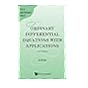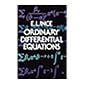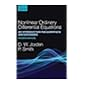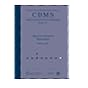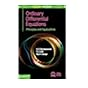515.352 HAS Classical methods in ordinary differential equations : with applications to boundary value problems / 515.352 HSU Ordinary differential equations with applications / 515.352 INC Ordinary differential equations. 515.352 JOR Nonlinear ordinary differential equations ; an introduction for scientists and engineers / 515.352 JOS Discrete Painleve equations / 515.352 NAN Ordinary differential equations : principles and applications / 515.352 NAN Ordinary differential equations : principles and applications /

Includes bibliographical references (p. -523) and index.